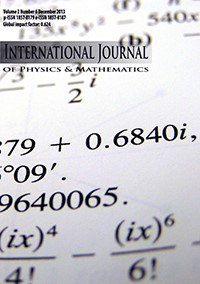International Journal of Physics & Mathematics (IJPM)

International Journal of Physics & Mathematics (IJPM) is a double-blind peer review online journal published annually by Association-Institute for English Language and American Studies (AIELAS), Tetovo - North Macedonia with the dedication to foster the latest advancements in Applied Physics and Mathematics. Generally, accepted papers will appear online within 8 months.

The aims and goals of International Journal of Physics and Mathematics (IJPM) are to provide a platform for scientists and academicians all over the world to promote, share, and discuss various new issues and developments in different areas of Applied Physics and Mathematics.

The scopes of the journal include, but are not limited to, the following research fields:

• Applications of Systems,
• Applied Mathematics,
• Applied Non-Linear Physics,
• Applied Optics,
• Applied Probability,
• Applied Solid State Physics,
• Applied Statistics,
• Approximation Theory,
• Biophysics,
• Chaos Theory,
• Combinatorics,
• Complexity Theory,
• Computability Theory,
• Computational Methods in Mechanics and Physics,
• Computational Physics,
• Condensed Matter Physics,
• Continuum Mechanics,
• Control Theory,
• Cryptography,
• Differentiable Dynamical Systems,
• Discrete Geometry,
• Dynamical Systems,
• Elastodynamics,
• Engineering and Industrial Physics,
• Evolutionary Computation,
• Experimental Mathematics,
• Financial Mathematics,
• Fluid Mechanics,
• Fuzzy Logic,
• Fuzzy Optimization,
• Game Theory,
• Geophysics,
• Graph Theory,
• Information Theory,
• Integrable Systems,
• Inverse Problems,
• Laser Physics,
• Linear Programming,
• Mathematical Biology,
• Mathematical Chemistry,
• Mathematical Economics,
• Mathematical Physics,
• Mathematical Psychology,
• Mathematical Sociology,
• Matrix Computations,
• Multi-Objective Optimization,
• Nanoscale Physics,
• Neural Networks,
• Nonlinear Partial Differential Equations,
• Non-Linear Physics,
• Nonlinear Processes in Physics,
• Nuclear Physics,
• Numerical Analysis,
• Numerical Computation,
• Operations Research,
• Optical Physics,
• Optimal Control,
• Optimization,
• Ordinary Differential Equations,
• Partial Differential Equations,
• Portfolio Selection,
• Probability Theory,
• Riemannian Manifolds,
• Scientific Computing,
• Set-Valued Analysis,
• Soliton Theory,
• Space Physics,
• Statistical Finance,
• Stochastic Processes,
• Symbolic Computation,
• Theoretical Statistics,
• Topological Dynamic Systems,
• Variational Inequality, and Vector Optimization.